# Julia Fractal set in C/C++ Using Graphics

The Julia set is associated with those points z = x + iy on the complex plane for which the series zn+1 = zn2 + c does not tend to infinity. c is a complex constant, one gets a different Julia set for each c. The initial value z0 for the series is each point in the image plane.

The well known Mandelbrot set forms a kind of index into the Julia set. A Julia set is either connected or disconnected, values of c chosen from within the Mandelbrot set are connected while those from the outside of the Mandelbrot set are disconnected. The disconnected sets are often called “dust”, they consist of individual points no matter what resolution they are viewed at.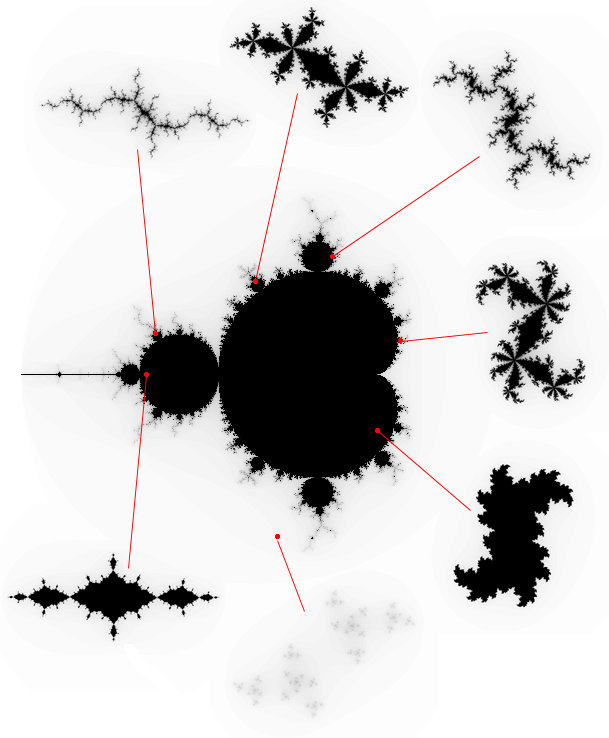### Code

 `#include ` `#include ` `#include ` `#include ` ` `  `#define Y 1079 ` `#define X 1919 ` ` `  `// To recursively find the end value ` `// of the passed point till the pixel ` `// goes out of the bounded region ` `// or the maximum depth is reached. ` `int` `julia_point(``float` `x, ``float` `y, ` `                ``int` `r, ``int` `depth, ` `                ``int` `max, ` `                ``double` `_Complex c, ` `                ``double` `_Complex z) ` `{ ` `    ``if` `(cabs(z) > r) { ` `        ``putpixel(x, y, ` `                 ``COLOR(255 - 255 * ((max - depth) * (max - depth)) % (max * max), ` `                       ``0, 0)); ` `        ``depth = 0; ` `    ``} ` `    ``if` `(``sqrt``(``pow``((x - X / 2), 2) ` `             ``+ ``pow``((y - Y / 2), 2)) ` `        ``> Y / 2) { ` `        ``putpixel(x, y, 0); ` `    ``} ` `    ``if` `(depth < max / 4) { ` `        ``return` `0; ` `    ``} ` `    ``julia_point(x, y, r, ` `                ``depth - 1, max, ` `                ``c, cpow(z, 2) + c); ` `} ` ` `  `// To select the points in a Julia set. ` `void` `juliaset(``int` `depth, ``double` `_Complex c, ``int` `r, ``int` `detail) ` `{ ` `    ``for` `(``float` `x = X / 2 - Y / 2; x < X / 2 + Y / 2; x += detail) { ` `        ``for` `(``float` `y = 0; y < Y; y += detail) { ` `            ``julia_point(x, y, r, ` `                        ``depth, depth, c, ` `                        ``(2 * r * (x - X / 2) / Y) ` `                            ``+ (2 * r * (y - Y / 2) / Y) ` `                                  ``* _Complex_I); ` `        ``} ` `    ``} ` `} ` ` `  `// Driver code ` `int` `main() ` `{ ` `    ``initwindow(X, Y); ` `    ``int` `depth = 100, r = 2, detail = 1; ` ` `  `    ``// Initial value for Julia ` `    ``// set taken by my personal preference. ` `    ``double` `_Complex c = 0.282 - 0.58 * _Complex_I; ` `    ``while` `(1) { ` ` `  `        ``cleardevice(); ` ` `  `        ``// To formulate the display text ` `        ``// for the 'c' coordinate ` `        ``// into string format. ` `        ``char` `str1, str2, strtemp; ` `        ``if` `(``floor``(creal(c)) == -1) { ` `            ``strcpy``(str1, ``"-0."``); ` `        ``} ` `        ``if` `(``floor``(creal(c)) == -0) { ` `            ``strcpy``(str1, ``"0."``); ` `        ``} ` `        ``if` `(``floor``(cimag(c)) == -1) { ` `            ``strcpy``(str2, ``"-0."``); ` `        ``} ` `        ``if` `(``floor``(cimag(c)) == -0) { ` `            ``strcpy``(str2, ``"0."``); ` `        ``} ` `        ``itoa(``sqrt``(``pow``(creal(c), 2)) * 1000, strtemp, 10); ` `        ``strcat``(str1, strtemp); ` `        ``strcat``(str1, ``", "``); ` `        ``itoa(``sqrt``(``pow``(cimag(c), 2)) * 1000, strtemp, 10); ` `        ``strcat``(str2, strtemp); ` `        ``strcat``(str1, str2); ` `        ``outtextxy(X * 0.8, Y * 0.8, str1); ` ` `  `        ``// To call the julia-set for the selected value of 'c'. ` `        ``juliaset(depth, c, r, detail); ` `        ``outtextxy(X / 3, Y * 0.9, ` `                  ``"Press '1' to Exit, Space to"` `                  ``" select a point or any "` `                  ``"other key to continue"``); ` `        ``char` `key = getch(); ` ` `  `        ``if` `(key == ``'\n'``) { ` `            ``break``; ` `        ``} ` ` `  `        ``// To select the value of 'c' ` `        ``// using the position of the mouse and then ` `        ``// normalizing it between a value of -1-1i and 1+1i. ` `        ``while` `(key == ``' '``) { ` ` `  `            ``c = 2 * (``double``)(mousex() - X / 2) / X + 2 * (mousey() - Y / 2) * _Complex_I / Y; ` ` `  `            ``if` `(``floor``(creal(c)) == -1) { ` `                ``strcpy``(str1, ``"-0."``); ` `            ``} ` ` `  `            ``if` `(``floor``(creal(c)) == -0) { ` `                ``strcpy``(str1, ``"0."``); ` `            ``} ` ` `  `            ``if` `(``floor``(cimag(c)) == -1) { ` `                ``strcpy``(str2, ``"-0."``); ` `            ``} ` ` `  `            ``if` `(``floor``(cimag(c)) == -0) { ` `                ``strcpy``(str2, ``"0."``); ` `            ``} ` ` `  `            ``itoa(``sqrt``(``pow``(creal(c), 2)) ` `                     ``* 1000, ` `                 ``strtemp, 10); ` `            ``strcat``(str1, strtemp); ` `            ``strcat``(str1, ``", "``); ` `            ``itoa(``sqrt``(``pow``(cimag(c), 2)) ` `                     ``* 1000, ` `                 ``strtemp, 10); ` `            ``strcat``(str2, strtemp); ` `            ``strcat``(str1, str2); ` `            ``outtextxy(X * 0.8, Y * 0.8, str1); ` `            ``if` `(kbhit()) { ` `                ``key = getch(); ` `            ``} ` `        ``} ` `    ``} ` `    ``closegraph(); ` `    ``return` `0; ` `} `

### Output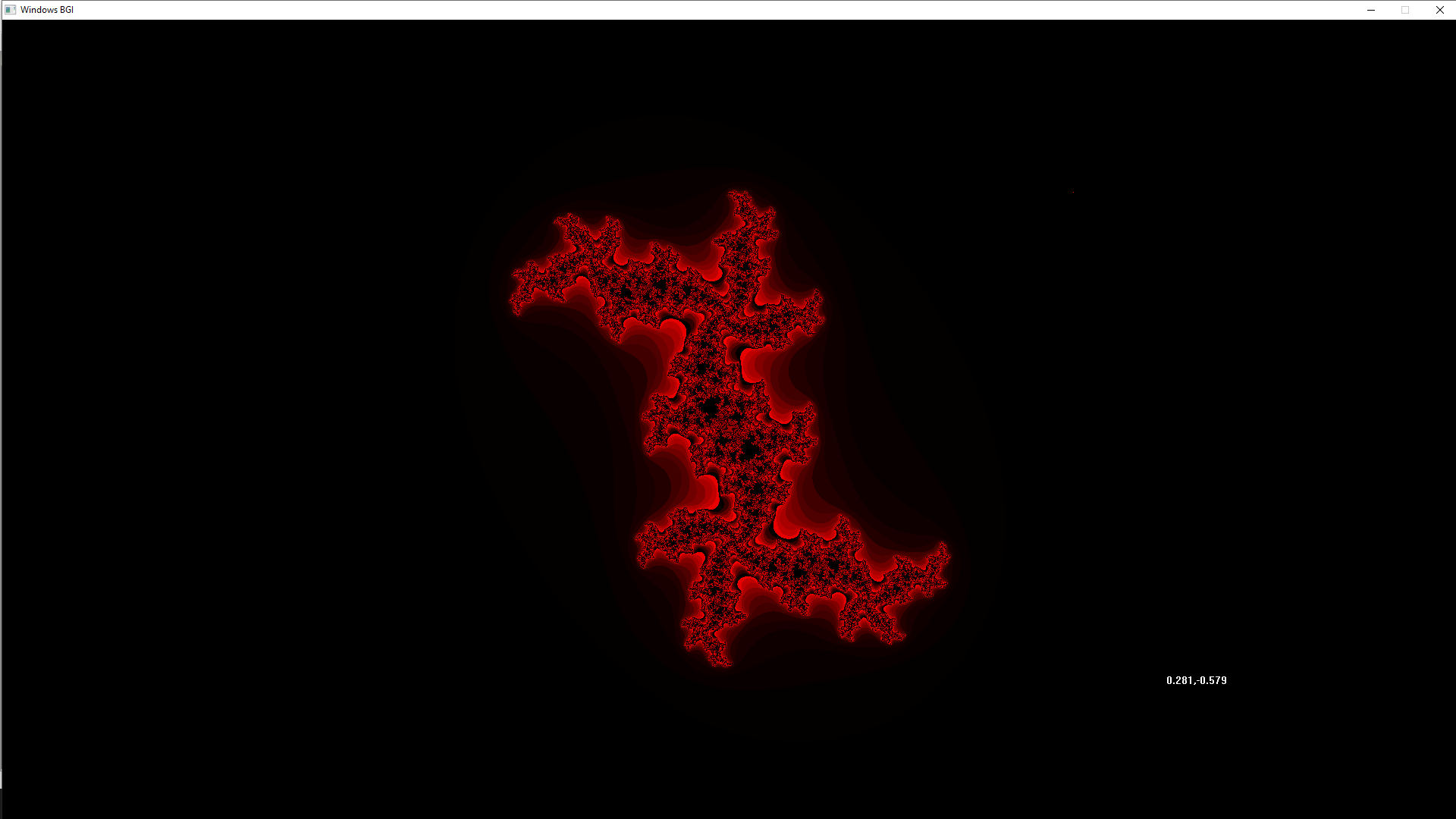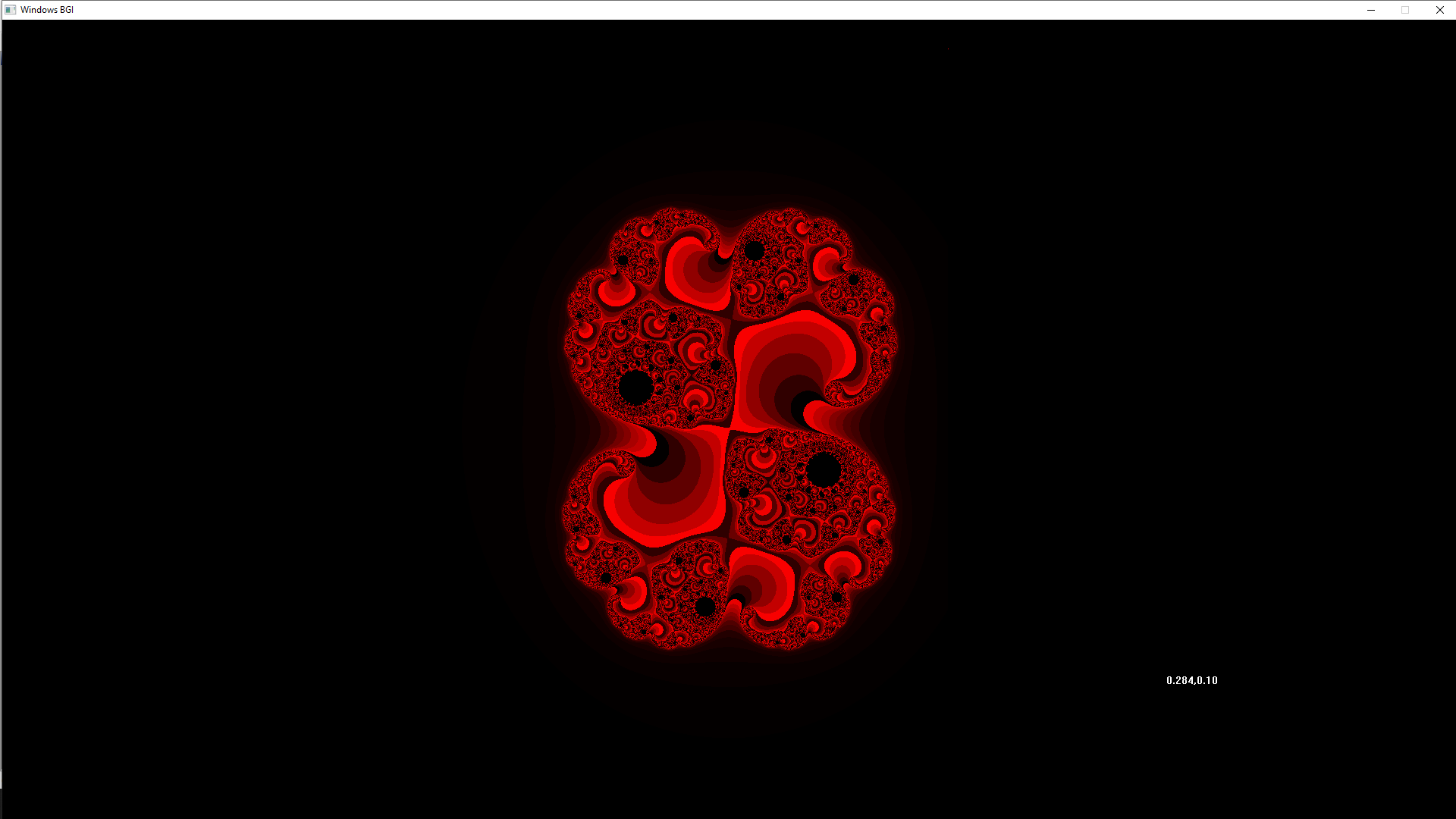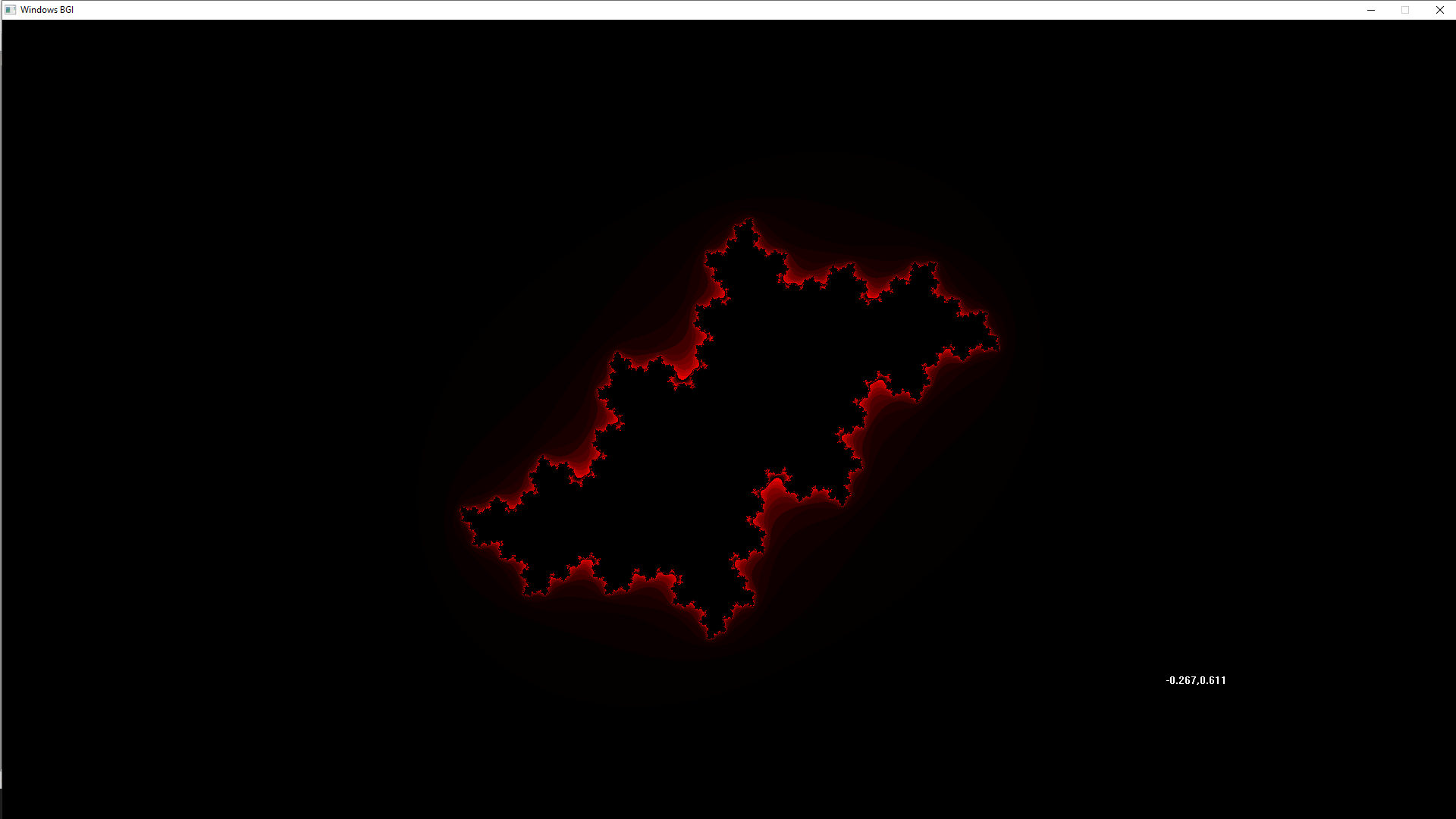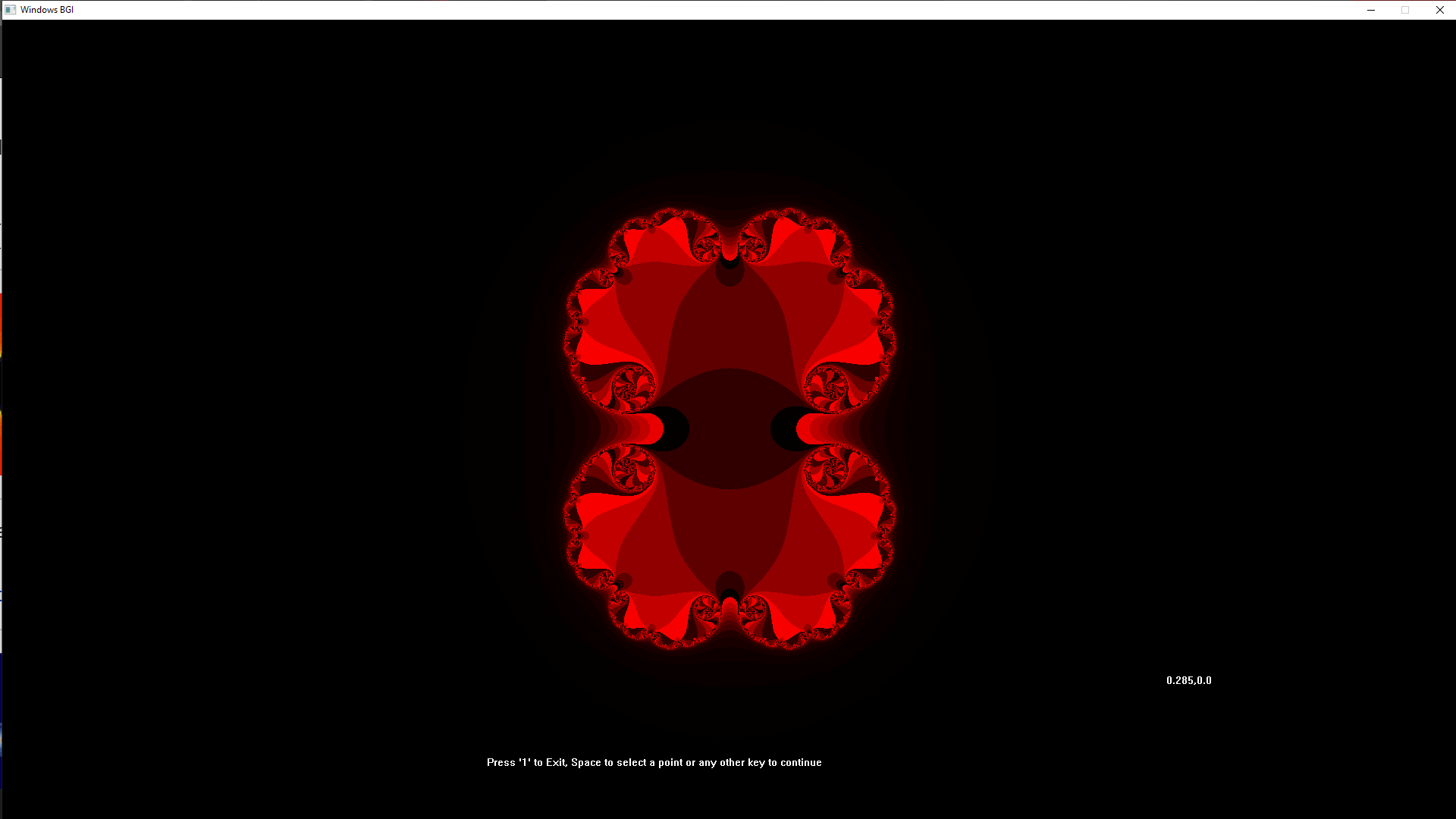My Personal Notes arrow_drop_upCheck out this Author's contributed articles.

If you like GeeksforGeeks and would like to contribute, you can also write an article using contribute.geeksforgeeks.org or mail your article to contribute@geeksforgeeks.org. See your article appearing on the GeeksforGeeks main page and help other Geeks.

Please Improve this article if you find anything incorrect by clicking on the "Improve Article" button below.

Article Tags :
Practice Tags :

Be the First to upvote.

Please write to us at contribute@geeksforgeeks.org to report any issue with the above content.